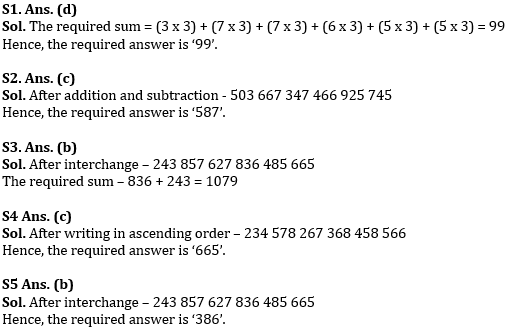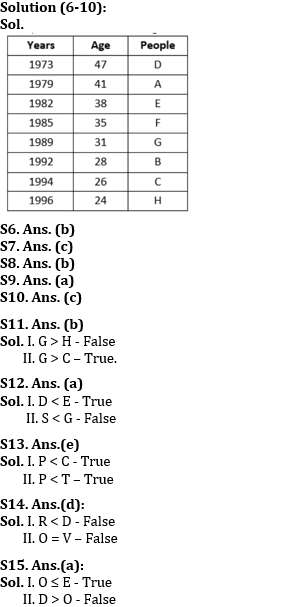Latest Banking jobs   »   Reasoning Ability Quiz For RBI Grade...

# Reasoning Ability Quiz For RBI Grade B/ ECGC PO/ SIDBI Grade A Prelims 2022- 28th April

Directions (1-5): Study the following data carefully and answer the questions accordingly.

423   587   267   386   845   665

Q1. If the last digit of each number is multiplied by 3 then what will be the sum of the resultant of all the digits after multiplication?
(a) 94
(b) 104
(c) 114
(d) 99
(e) None of these

Q2. If we subtract 2 from the second digit of each number and add 1 to the first digit of each number, then which of the following will be the third highest number?
(a) 423
(b) 665
(c) 587
(d) 386
(e) None

Q3. If the first two digits of each number are interchanged then what will be the resultant of the sum of the lowest and the second highest number?
(a) 1132
(b) 1079
(c) 1010
(d) 1099
(e) None of these

Q4. If all the digits in each number are written in ascending order within the number, then which of the given number will become the second highest?
(a) 587
(b) 386
(c) 665
(d) 845
(e) None of these

Q5. If the first two digits of each number are interchanged then which of the following number will be second highest?
(a) 267
(b) 386
(c) 423
(d) 587
(e) None of these

Directions (6-10): Study the following information carefully and answer the given questions.
Eight people i.e. A, B, C, D, E, F, G and H were born in different years i.e., 1996, 1973, 1979, 1985, 1994, 1992, 1982 and 1989 but not necessarily in the same order. (Note: Consider the base year as 2020.) The age of B is a multiple of 7. Three people were born between F and H. One person was born between B and F who was born before B. The difference between the age of H and C is 2 years. Two people were born between G and A who is not the youngest. D was not born after E. The difference between the age of C and G is 5 years.

Q6. Who amongst the following is the oldest person?
(a) G
(b) D
(c) F
(d) H
(e) None of these

Q7. How many people were born between A and the one who was born just after G?
(a) Four
(b) Two
(c) Three
(d) Five
(e) None of these

Q8. What is the age of the one who was born just before E?
(a) 38 years
(b) 41 years
(c) 35 years
(d) 28 years
(e) None of these

Q9. Four of the following five are alike in a certain way and hence form a group. Which is the one that doesn’t belong to that group?
(a) D
(b) E
(c) B
(d) C
(e) H

Q10. Who amongst the following was born in 1992?
(a) H
(b) D
(c) B
(d) E
(e) None of these

Directions (11-15): In each of the following questions, assuming the given statements to be true, find which of the following options holds true:

Q11. Statements: F ≥ H, A ≤ C, G ≥ H, H > C
Conclusions:
I. G > H
II. G > C

(a) If only conclusion I is true.
(b) If only conclusion II is true.
(c) If either conclusion I or conclusion II is true.
(d) If neither conclusion I nor conclusion II is true
(e) If both conclusions I and II are true.

Q12. Statements: J ≤ K, S > D < T, E ≥ S, E = G
Conclusions:
I. D < E
II. S < G

(a) If only conclusion I is true.
(b) If only conclusion II is true.
(c) If either conclusion I or conclusion II is true.
(d) If neither conclusion I nor conclusion II is true
(e) If both conclusions I and II are true.

Q13. Statements: T ≥ M, M < K, C = K, P < M
Conclusions:
I. P < C
II. P < T

(a) If only conclusion I is true.
(b) If only conclusion II is true.
(c) If either conclusion I or conclusion II is true.
(d) If neither conclusion I nor conclusion II is true
(e) If both conclusions I and II are true.

Q14. Statements: R > S < O ≥ C, S = V > D ≥ A
Conclusions:
I. R < D
II. O = V

(a) If only conclusion I is true.
(b) If only conclusion II is true.
(c) If either conclusion I or conclusion II is true.
(d) If neither conclusion I nor conclusion II is true
(e) If both conclusions I and II are true.

Q15. Statements: D < E ≥ C = V > G ≤ T, C ≥ O
Conclusions:
I. O ≤ E
II. D > O

(a) If only conclusion I is true.
(b) If only conclusion II is true.
(c) If either conclusion I or conclusion II is true.
(d) If neither conclusion I nor conclusion II is true
(e) If both conclusions I and II are true.

Solutions